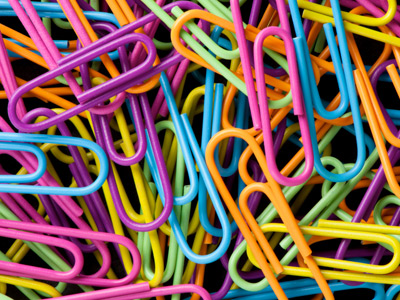How many paper clips would you expect to find in a box labeled 'average contents 100'?

# Data Handling - Averages 2

This Math quiz is called 'Data Handling - Averages 2' and it has been written by teachers to help you if you are studying the subject at middle school. Playing educational quizzes is a fabulous way to learn if you are in the 6th, 7th or 8th grade - aged 11 to 14.

It costs only \$12.50 per month to play this quiz and over 3,500 others that help you with your school work. You can subscribe on the page at Join Us

Mean and mode are two types of averages found in data handling. An average is a typical or representative value of a data set.

See how much you know about the subject in the following Math quiz which deals with the three averages - mean, mode and median - which can be used in different situations.

1.
A box of paper clips is labeled 'average contents 100'. How many paper clips would you expect to find in the box?
100
At least 100
More than 100
You might get 102 in one box and 98 in another, but the contents should always be about 100
2.
Which of these is always one of the data values in a set?
Mean
Median
Mode
Range
Remember, the mode is the most common
3.
Of 20 customers at a vending machine, 8 spent 50p, 5 spent 60p, 4 spent 75p and 3 spent £1. What was the total amount spent?
£2.85
£8.50
£10.90
£13.00
(8 x 0.50) + (5 x 0.60) + (4 x 0.75) + (3 x 1)
4.
What is the modal amount spent by these 20 customers?
50p
60p
75p
£1
Modal amount = mode (most frequent)
5.
What is the median of this data?
50p
60p
75p
£1
Both the 10th and 11th values are 60p
6.
To find the mean of a data set, add up all values then divide by the .......
number of values in the set
biggest number in the set
smallest value in the set
middle value of the set
7.
What is the mean amount spent by the 20 vending machine customers?
50p
55p
60p
65p
Mean = total ÷ 20. £13.00 ÷ 20 = 0.65
8.
Which averages could best represent this set of data?
Mode and median
Mean and mode
Median and mean
Any of them
The mean is higher than the amount spent by most customers. 13 of them spent 60p or less
9.
The mean length of calls to a help desk is 3 minutes. If the advisers take 1,000 calls, how much time do they spend on the 'phone?
20 hours
30 hours
40 hours
50 hours
1,000 x 3 = 3,000 minutes. Divide by 60 to convert to hours
10.
The mean of the first 9 calls is 5 minutes. The next call takes 10 minutes. What is the mean of the first 10 calls?
4.5 minutes
5 minutes
5.5 minutes
6 minutes
Total call time is (9 x 5) + 10 = 55 so mean is 55 ÷ 10 = 5.5
Author:  Frank Evans